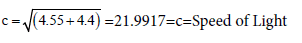The Meaning of and#8220;Piand#8221; in the Hebrew Scriptures | OMICS International
Journal of Physical Mathematics
All submissions of the EM system will be redirected to Online Manuscript Submission System. Authors are requested to submit articles directly to Online Manuscript Submission System of respective journal.

# The Meaning of “Pi” in the Hebrew Scriptures

Cusak P*

*Corresponding Author:
Cusak P
Tel: 01191258945
E-mail: [email protected]

Received Date: June 15, 2016; Accepted Date: August 22, 2016; Published Date: August 25, 2016

Citation: Cusak P (2016) The Meaning of “Pi” in the Hebrew Scriptures. J Phys Math 7: 195. doi: 10.4172/2090-0902.1000195

Copyright: © 2016 Cusak P. This is an open-access article distributed under the terms of the Creative Commons Attribution License, which permits unrestricted use, distribution, and reproduction in any medium, provided the original author and source are credited.

Visit for more related articles at Journal of Physical Mathematics

#### Short Commentary

The Ancient Physicists knew far more Physics than scientists of today, until now. This paper shows briefly, how the Hebrews recorded this advanced cosmology. The following calculations show how the Hebrews included these calculations in an encrypted way. That the ratio of the circumference to the diameter of a circle is constant (namely, pi) has been recognized for as long as we have written records [1,2].

And he made a molten sea, ten cubits from the one brim to the other: it was round all about, and his height was five cubits: and a line of thirty cubits did compass it about. [3,4]

10 cubits=4.4~4.482=Mass

5 cubits=1385 (1/13.85)13.85 or 86.16 (1/86.2=116 elements in the Periodic Table of the Elements)

5 x 44=220 1/220=455

(455+4.4)/2=4.475~4.486=Mass

4.55+4.4=c2=Speed of Light squared

30 cubits=1320 1320/3.281=402.3=Reynold's Number

13.20-100=868=sin 60 degrees=Force=Energy

So, E=Mc2 ("Einstein's" Equation)

E=4.475* 8.95=4.00=(2(E+t)=2(1+1)=4 Vector Space

E=0.868=sin 60.2 degrees (Energy and Time Vectors, Sexigesimal System)4/2.9917=1.337~4/3=Space

Energy:

(0.1440+0.1385+0.1320)/2.9917=0.1385

0.8615 168

F=4.475/168=2.667=8/3=Superforce

sin-1(0.26637)=0.1544 0.8455 57.72 degrees

1/ 2.42=0.4132 0.4132-3.125 (Babylonian Pi)=2.7118=Base e

10/5/30=1.0530

3.125 (Pi)/ 1.0530=2.967~c (recall)

p2/(2M)+v=E

v=6.68~~G=Gravitational Constant

The 11 Dimensions of the universe are from the Interest formula (1+i)N.

#### References

Select your language of interest to view the total content in your interested language

### Article Usage

• Total views: 8618
• [From(publication date):
September-2016 - Nov 19, 2019]
• Breakdown by view type
• HTML page views : 8513Can't read the image? click here to refresh